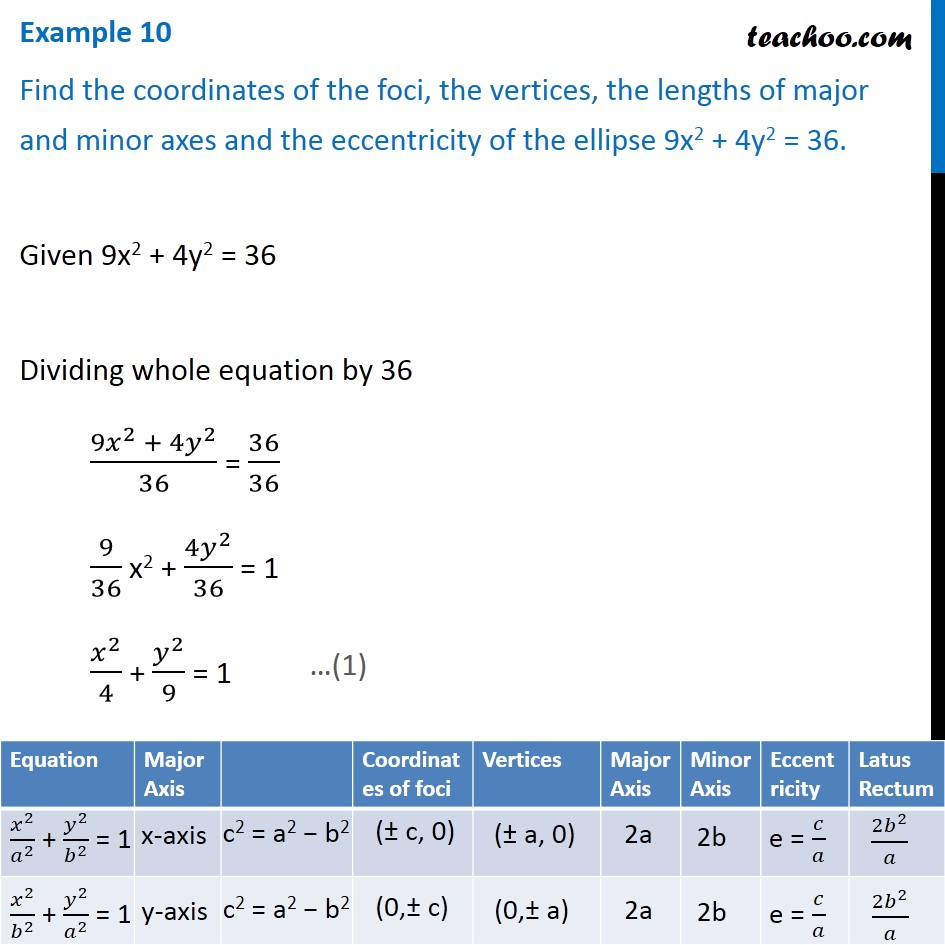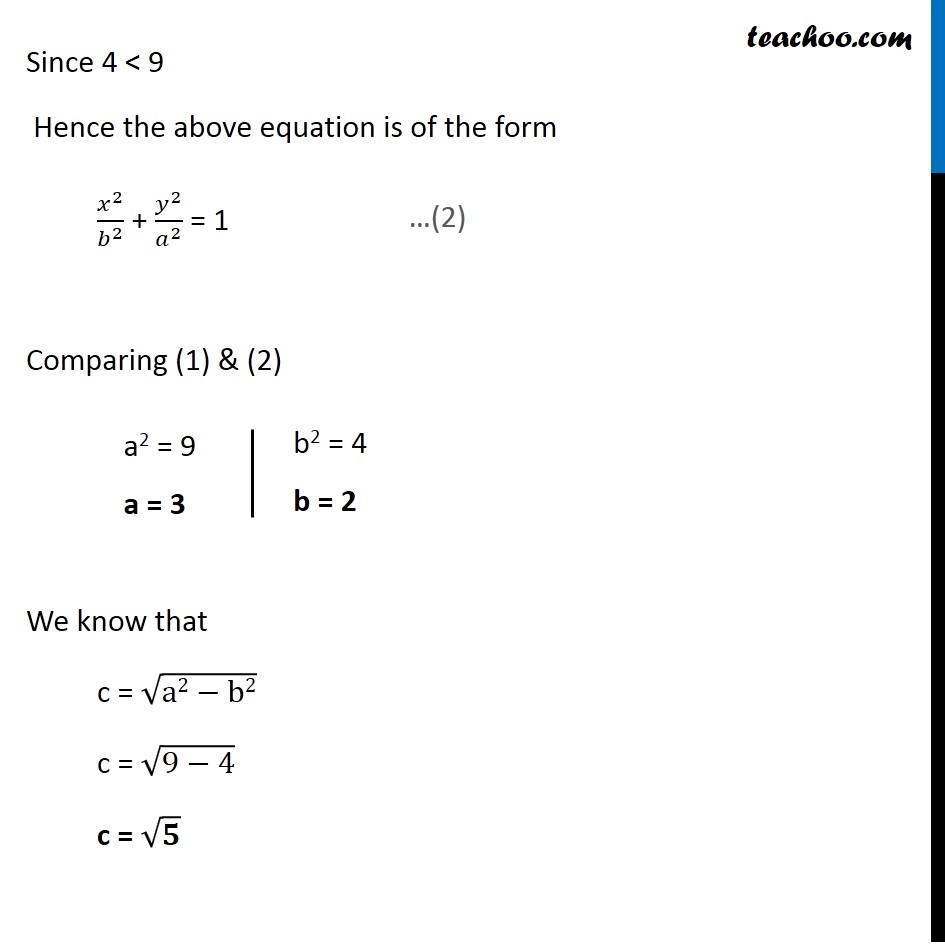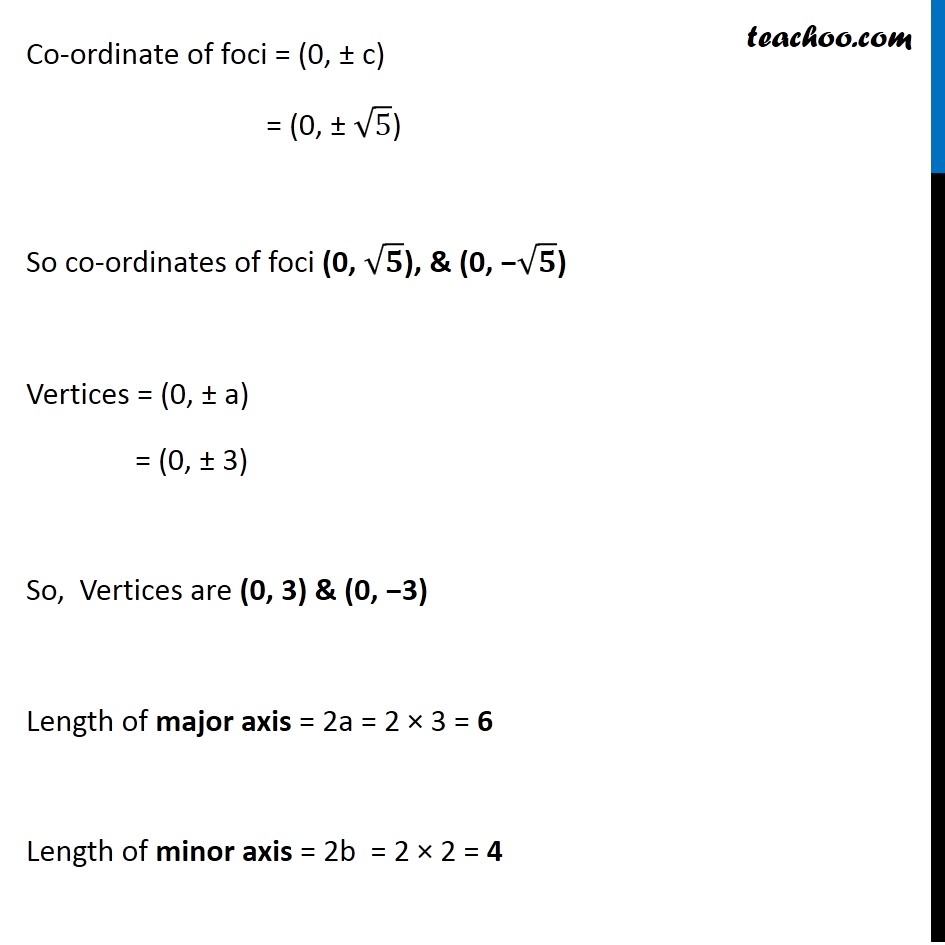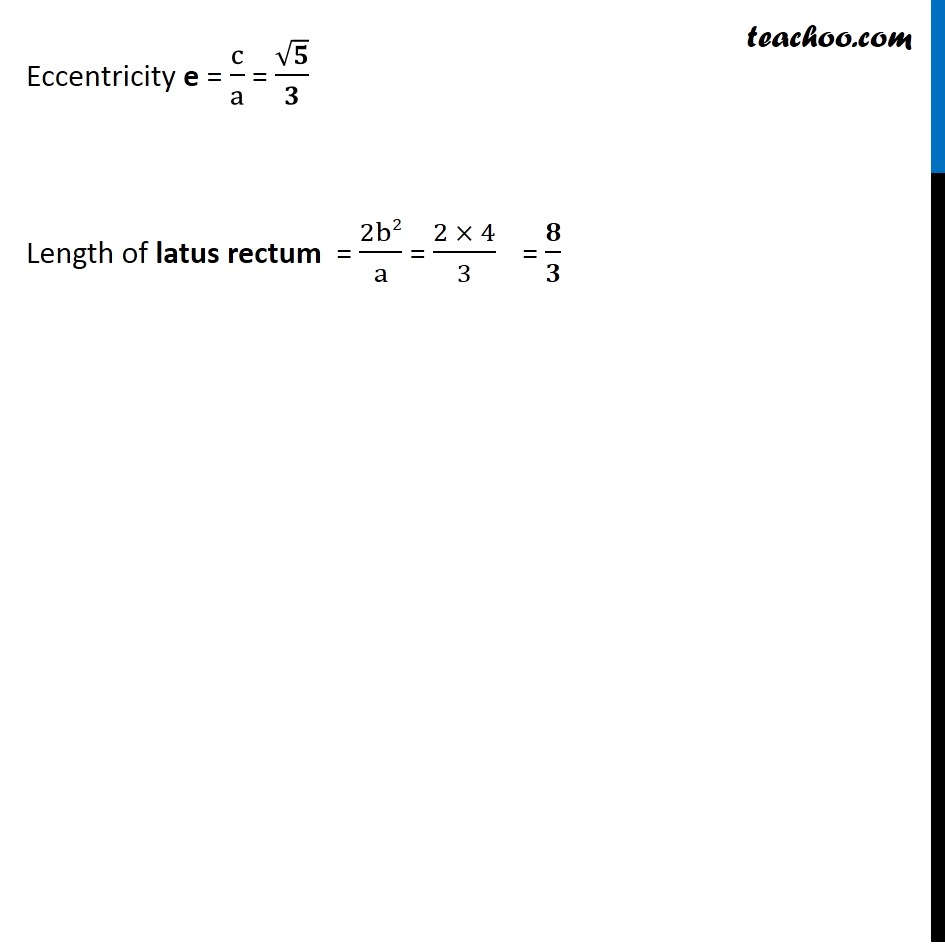Ellipse - Defination

Chapter 10 Class 11 Conic Sections
Concept wiseLearn in your speed, with individual attention - Teachoo Maths 1-on-1 Class

### Transcript

Example 10 Find the coordinates of the foci, the vertices, the lengths of major and minor axes and the eccentricity of the ellipse 9x2 + 4y2 = 36. Given 9x2 + 4y2 = 36 Dividing whole equation by 36 (9𝑥^2 + 4𝑦^2)/36 = 36/36 9/36 x2 + (4𝑦^2)/36 = 1 𝑥^2/4 + 𝑦^2/9 = 1 Since 4 < 9 Hence the above equation is of the form 𝑥^2/𝑏^2 + 𝑦^2/𝑎^2 = 1 Comparing (1) & (2) We know that c = √(a2−b2) c = √(9−4) c = √𝟓 Co-ordinate of foci = (0, ± c) = (0, ± √5) So co-ordinates of foci (0, √𝟓), & (0, −√𝟓) Vertices = (0, ± a) = (0, ± 3) So, Vertices are (0, 3) & (0, −3) Length of major axis = 2a = 2 × 3 = 6 Length of minor axis = 2b = 2 × 2 = 4 Eccentricity e = c/a = √𝟓/𝟑 Length of latus rectum = 2b2/a = (2 × 4)/3 = 𝟖/𝟑